# Difference between revisions of "Bieberbach-Eilenberg functions"

in the disc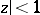The class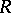of functions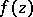, regular in the disc, which have an expansion of the form(1)

and which satisfy the condition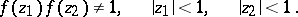This class of functions is a natural extension of the classof functions, regular in the disc, with an expansion (1) and such that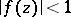for. The class of univalent functions (cf. Univalent function) inis denoted by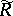. The functions inwere named after L. Bieberbach , who showed that for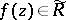the inequality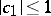(2)

is valid, while equality holds only for the function, whereis real, and after S. Eilenberg , who proved that the inequality (2) is valid for the whole class. It was shown by W. Rogosinski  that every function inis subordinate (cf. Subordination principle) to some function in. Inequality (2) yields the following sharp inequality for: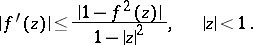(3)

The following bound on the modulus of a function inhas been obtained: If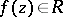, then(4)

and (4) becomes an equality only for the functions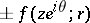, whereis real andThe method of the extremal metric (cf. Extremal metric, method of the) provided the solution of the problem of the maximum and minimum of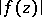in the classof functions inwith a fixed value,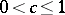, in the expansion (1): For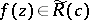,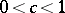, the following sharp inequalities are valid:(5)

Here the functionsand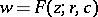map the disconto domains which are symmetric with respect to the imaginary axis of the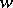-plane, and the boundaries of which belong to the union of the closures of certain trajectories or orthogonal trajectories of a quadratic differential in the-plane with a certain symmetry in the distribution of the zeros and poles , . Certain optimal results for functions in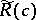were obtained by the simultaneous use of the method of the extremal metric and the symmetrization method .

Many results obtained for the functions in the classesandare consequences of corresponding results for systems of functions mapping the disconto disjoint domains . The analogue offor a finitely-connected domainwithout isolated boundary points and not containing the point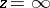, is the class,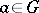, of functionsregular inand satisfying the conditions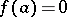,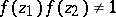, whereare arbitrary points in. The classextends the classof functions, regular inand such that,in. The following sharp estimate is an extension of the result of Bieberbach–Eilenberg and of inequality (3) to functions of class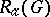: If, then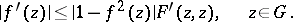where, is that function infor which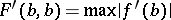in this class.

How to Cite This Entry:
Bieberbach-Eilenberg functions. Encyclopedia of Mathematics. URL: http://encyclopediaofmath.org/index.php?title=Bieberbach-Eilenberg_functions&oldid=16091
This article was adapted from an original article by G.V. Kuz'mina (originator), which appeared in Encyclopedia of Mathematics - ISBN 1402006098. See original article